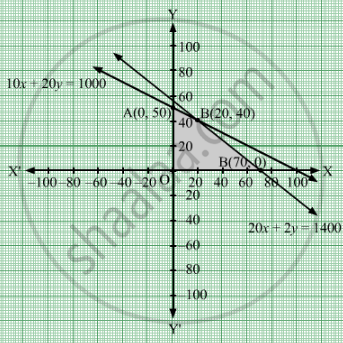# A Man Owns a Field of Area 1000 Sq.M. He Wants to Plant Fruit Trees in It. He Has a Sum of Rs 1400 to Purchase Young Trees. - Mathematics

Sum

A man owns a field of area 1000 sq.m. He wants to plant fruit trees in it. He has a sum of Rs 1400 to purchase young trees. He has the choice of two types of trees. Type A requires 10 sq.m of ground per tree and costs Rs 20 per tree and type B requires 20 sq.m of ground per tree and costs Rs 25 per tree. When fully grown, type A produces an average of 20 kg of fruit which can be sold at a profit of Rs 2.00 per kg and type B produces an average of 40 kg of fruit which can be sold at a profit of Rs. 1.50 per kg. How many of each type should be planted to achieve maximum profit when the trees are fully grown? What is the maximum profit?

#### Solution

Let the man planted x trees of type A and y trees of type B.
Number of trees cannot be negative.
Therefore, $x, y \geq 0$ To plant tree of type A requires 10 sq.m and type B requires 20 sq.m of ground per tree. And, it is given that a man owns a field of area 1000 sq.m.Therefore,

$10x + 20y \leq 1000$

Type A costs Rs 20 per tree and type B costs Rs 25 per tree. Therefore, x trees of type Aand y trees of type costs Rs 20x and Rs 25y respectively. A man has a sum of Rs 1400 to purchase young trees.
$20x + 25y \leq 1400$
Thus, the mathematical formulat​ion of the given linear programmimg problem is
Max Z =  40− 20+ 60− 25y = 20x + 35y
subject to

$10x + 20y \leq 1000$
$20x + 25y \leq 1400$

The feasible region determined by the system of constraints isThe corner points are A(0, 50), B(20, 40), C(70, 0)

The values of Z at these corner points are as follows

 Corner point Z = 20x + 35y A 1750 B 1800 C 1400

The maximum value of Z is 1800 which is attained at B(20, 40)
Thus, the maximum profit is Rs 1800 obtained when Rs 20 were invested on type A and Rs 40 were invested on type B.

Concept: Graphical Method of Solving Linear Programming Problems
Is there an error in this question or solution?

#### APPEARS IN

RD Sharma Class 12 Maths
Chapter 30 Linear programming
Exercise 30.4 | Q 25 | Page 53